# 第七章 正则化(Regularization)

## 7.1 过拟合的问题

• 到现在为止 你已经见识了 几种不同的学习算法 包括线性回归和逻辑回归 它们能够有效地解决许多问题 但是当将它们应用到 某些特定的机器学习应用时 会遇到过度拟合(over-fitting)的问题 可能会导致它们效果很差

• 在这段视频中 我将为你解释 什么是过度拟合问题 并且 在此之后接下来的几个视频中 我们将谈论一种 称为正则化(regularization)的技术 它可以改善或者 减少过度拟合问题 以使学习算法更好实现 那么什么是过度拟合呢？

• 让我们继续使用 那个用线性回归 来预测房价的例子 我们通过建立 以住房面积为自变量的函数来预测房价 我们可以 对该数据做线性回归 如果这么做 我们也许能够获得 拟合数据的这样一条直线 但是 这不是一个很好的模型 我们看看这些数据 很明显 随着房子面积增大 住房价格的变化趋于稳定 或者越往右越平缓 因此该算法 没有很好拟合训练数据 我们把这个问题称为欠拟合(underfitting) 这个问题的另一个术语叫做 高偏差(High bias) 这两种说法大致相似 意思是它只是没有很好地拟合训练数据 这个词是 过去传下来的一个专业名词 它的意思是 如果拟合一条直线 到训练数据 就好像算法 有一个很强的偏见 或者说非常大的偏差 因为该算法认为房子价格与面积仅仅线性相关 尽管与该数据的事实相反 尽管相反的证据 被事前定义为 偏差 它还是接近于 拟合一条直线 而此法最终导致拟合数据效果很差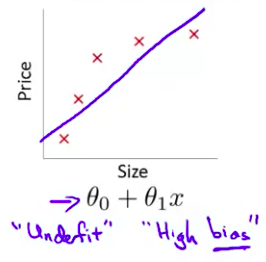• 我们现在可以在中间 加入一个二次项 在这组数据中 我们用二次函数来拟合它 然后可以拟合出一条曲线 事实证明这个拟合效果很好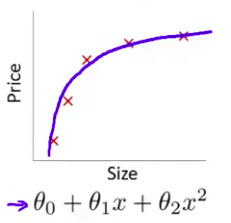• 另一个极端情况是 如果我们拟合一个四次多项式 因此在这里我们有五个参数 $\theta_0$到$\theta_4$ 这样我们可以拟合一条曲线 通过我们的五个训练样本 你可以得到看上去如此的一条曲线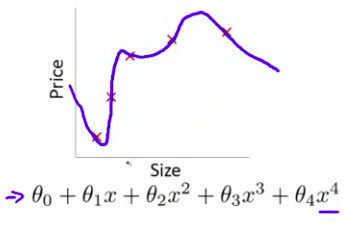• 一方面 似乎 对训练数据 做了一个很好的拟合 因为这条曲线通过了所有的训练实例 但是 这仍然是一条扭曲的曲线 对吧？ 它不停上下波动 因此事实上 我们并不认为它是一个预测房价的好模型 所以 这个问题我们把他叫做 过度拟合过拟合(overfitting) 另一个描述该问题的术语是 高方差(variance) 高方差是另一个 历史上的叫法 但是 从第一印象上来说 如果我们拟合一个 高阶多项式 那么 这个函数能很好的拟合训练集 能拟合几乎所有的 训练数据 这就面临可能函数太过庞大的问题 变量太多 同时如果我们没有足够的数据 去约束这个变量过多的模型 那么这就是过度拟合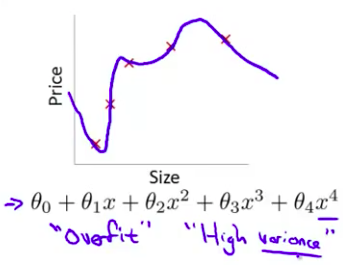• 在两者之间的情况 叫”刚好合适” 这并不是一个真正的名词 我只是把它写在这里 这个二次多项式 二次函数 可以说是恰好拟合这些数据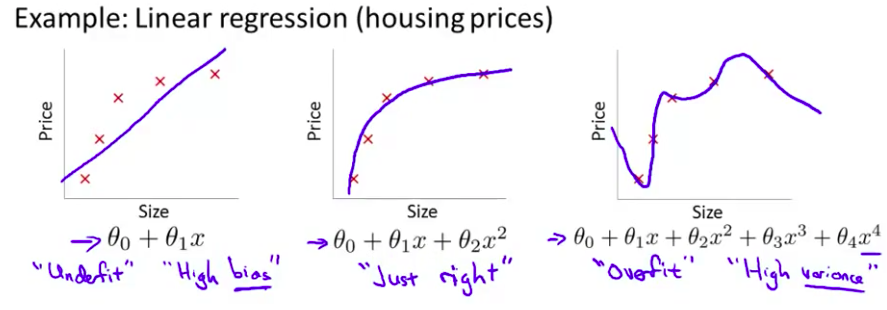• 概括地说 过度拟合的问题 将会在变量过多的时候 发生 这种时候训练出的方程总能很好的拟合训练数据 所以 你的代价函数 实际上可能非常接近于0 或者 就是0 但是 这样的曲线 它千方百计的拟合于训练数据 这样导致 它无法泛化到 新的数据样本中 以至于无法预测新样本价格 在这里 术语”泛化“ 指的是一个假设模型能够应用到新样本的能力 新样本数据是 没有出现在训练集中的房子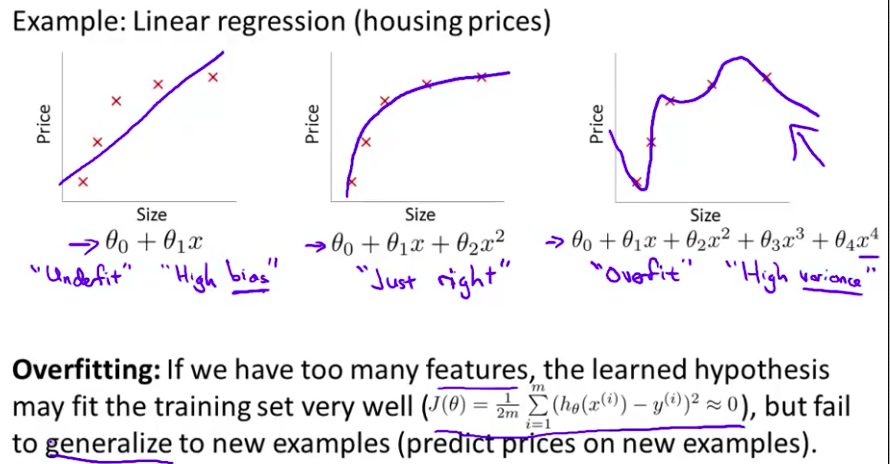• 在这张幻灯片上 我们看到了 线性回归情况下的过拟合 类似的方法同样可以应用到逻辑回归 这里是一个以x1与x2为变量的 逻辑回归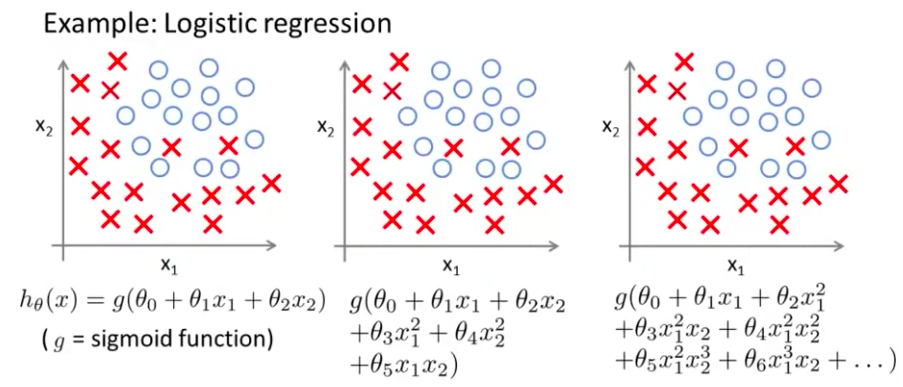• 我们可以做的就是 用这样一个简单的假设模型 来拟合逻辑回归 和以前一样 字母$g$代表S型函数 如果这样做 你会得到一个假设模型 这个假设模型是一条直线 它直接分开了正样本和负样本 但这个模型并不能够很好的拟合数据 因此 这又是一个欠拟合的例子 或者说假设模型具有高偏差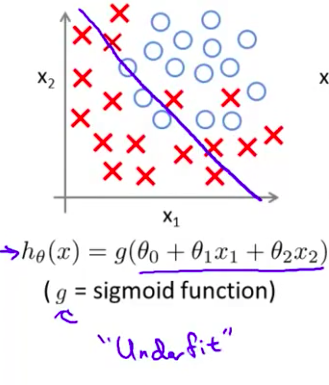• 相比之下 如果 如果再加入一些变量 比如这些二次项 那么你可以得到一个判定边界 像这样 这样就很好的拟合了数据 这很可能 是训练集的最好拟合结果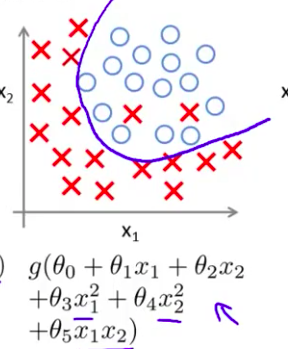• 最后 在另一种极端情况下 如果你用高阶多项式来拟合数据 你加入了很多 高阶项 那么逻辑回归可能发生自身扭曲 它千方百计的 形成这样一个 判定边界 来拟合你的训练数据 以至于成为一条扭曲的曲线 使其能够拟合每一个训练集中的样本 而且 如果x1和x2 能够预测 癌症 你知道 癌症是一种恶性肿瘤 同时肿瘤也可能是良性 确实 这个假设模型不是一个很好的预测 因此 这又是一个过拟合例子 是一个 有高方差的假设模型 并且不能够很好泛化到新样本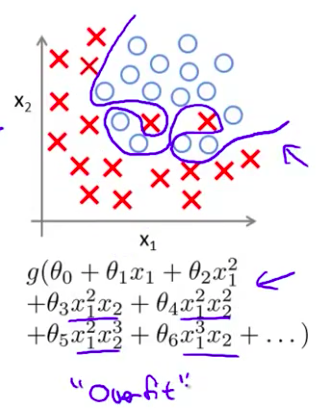• 在今后课程中 我们会讲到调试和诊断 诊断出导致学习算法故障的东西 我们告诉你如何用 专门的工具来识别 过拟合 和可能发生的欠拟合 但是 现在 让我们谈谈 过拟合 的问题 我们怎么样解决呢
• 在前面的例子中 当我们使用一维或二维数据时 我们可以通过绘出假设模型的图像来研究问题所在 再选择合适的多项式来拟合数据 因此 以之前的房屋价格为例 我们可以 绘制假设模型的图像 就能看到 模型的曲线 非常扭曲并通过所有样本房价 我们可以通过绘制这样的图形 来选择合适的多项式阶次 因此绘制假设模型曲线 可以作为决定多项式阶次 的一种方法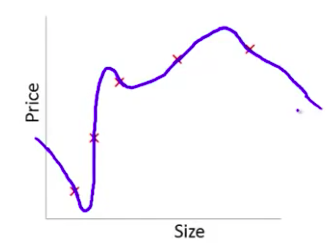• 但是这并不是总是有用的 而且事实上更多的时候我们 会遇到有很多变量的假设模型 并且 这不仅仅是选择多项式阶次的问题 事实上 当我们 有这么多的特征变量 这也使得绘图变得更难 并且 更难使其可视化 因此并不能通过这种方法决定保留哪些特征变量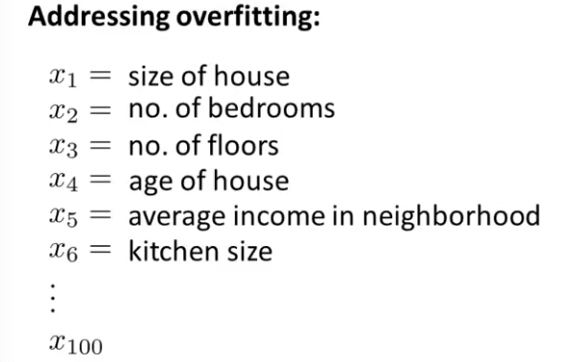• 具体地说 如果我们试图 预测房价 同时又拥有这么多特征变量 这些变量看上去都很有用 但是 如果我们有 过多的变量 同时 只有非常少的训练数据 就会出现过度拟合的问题
• 为了解决过度拟合 有两个办法 来解决问题 第一个办法是要尽量 减少选取变量的数量 具体而言 我们可以人工检查 变量的条目 并以此决定哪些变量更为重要 然后 决定保留哪些特征变量 哪些应该舍弃
• 在今后的课程中 我们会提到模型选择算法 这种算法是为了自动选择 采用哪些特征变量 自动舍弃不需要的变量 这种减少特征变量 的做法是非常有效的 并且可以减少过拟合的发生 当我们今后讲到模型选择时 我们将深入探讨这个问题 但是其缺点是 舍弃一部分特征变量 你也舍弃了 问题中的一些信息 例如 也许所有的 特征变量 对于预测房价都是有用的 我们实际上并不想 舍弃一些信息 或者舍弃这些特征变量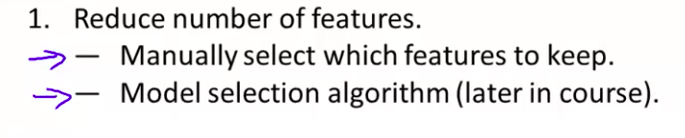• 第二个选择 我们将在接下来的视频中讨论 就是正则化regularization 正则化中我们将保留 所有的特征变量 但是减少参数 $\theta_j$ 的大小 这个方法非常有效
• 当我们有很多特征变量时 其中每一个变量 都能对预测产生一点影响 y的值 正如我们在房价的例子中看到的那样 在那里我们可以有很多特征变量 其中每一个变量 都是有用的 因此我们不希望把它们删掉 这就导致了 正则化概念的发生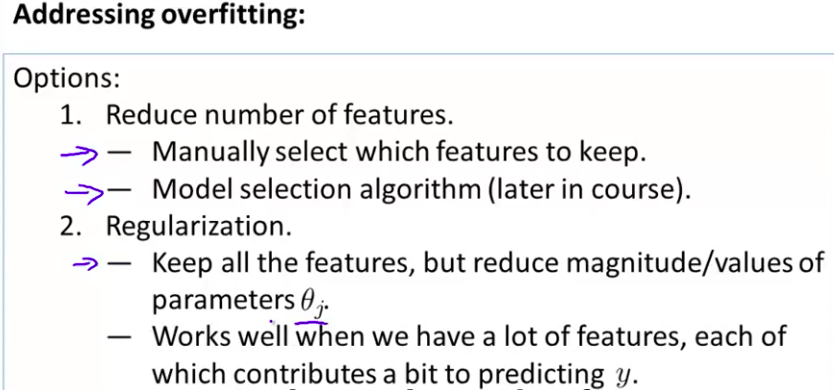• 我知道 这些东西你们现在可能还听不懂 但是在接下来的视频中 我们将开始详细讲述 怎样应用正则化和什么叫做正则化均值 然后我们将开始 讲解怎样使用正则化 怎样使学习算法正常工作 并避免过拟合

### 小小的总结–接下来是别人的笔记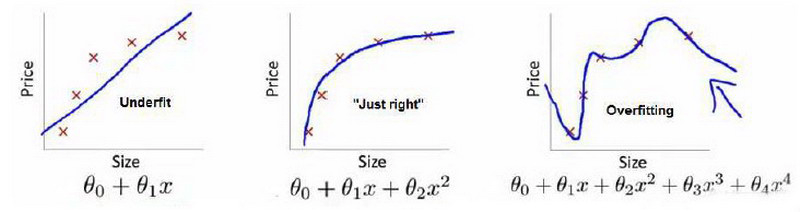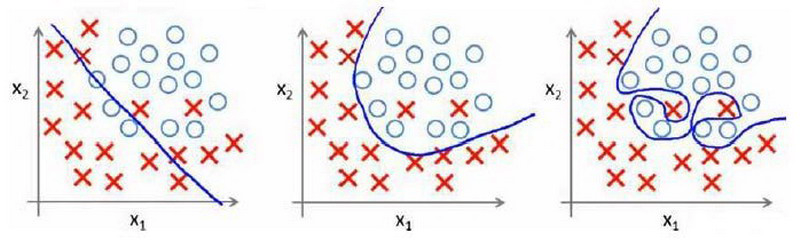1. 丢弃一些不能帮助我们正确预测的特征。可以是手工选择保留哪些特征，或者使用一些模型选择的算法来帮忙（例如PCA）

2. 正则化。 保留所有的特征，但是减少参数$\theta_j$的大小（magnitude）。

## 7.2 代价函数

• 在这段视频中 传达给你一个直观的感受 告诉你正规化是如何进行的 而且 我们还要写出 我们使用正规化时 需要使用的代价函数 根据我们幻灯片上的 这些例子 我想我可以给你一个直观的感受 但是 一个更好的 让你自己去理解正规化 如何工作的方法是 你自己亲自去实现它 并且看看它是如何工作的 如果在这节课后 你进行一些适当的练习 你就有机会亲自体验一下 正规化到底是怎么工作的 那么 这里就是一些直观解释
• 在前面的视频中 我们看到了 如果说我们要 用一个二次函数来 拟合这些数据 它给了我们一个对数据很好的拟合 然而 如果我们 用一个更高次的 多项式去拟合 我们最终 可能得到一个曲线 能非常好地拟合训练集 但是 这真的不是一个好的结果 它过度拟合了数据 因此 一般性并不是很好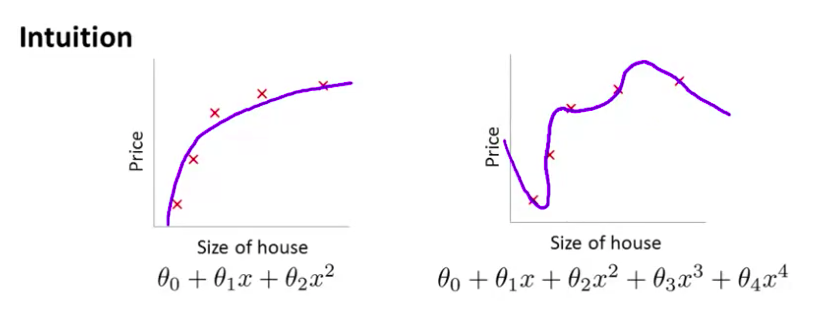• 让我们考虑下面的假设 我们想要加上惩罚项 从而使 参数 $\theta_3$ 和 $\theta_4$ 足够的小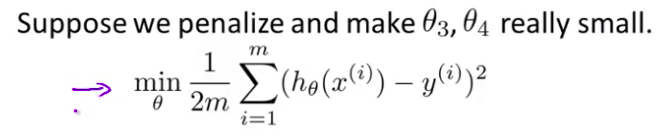• 这里我的意思就是 这是我们的优化目标 或者客观的说 这就是我们需要 优化的问题 我们需要尽量减少 代价函数的均方误差 对于这个函数 我们对它进行一些 添加一些项 加上 1000 乘以 $\theta_3$ 的平方 再加上 1000 乘以 $\theta_4$ 的平方 1000 只是我随便写的某个较大的数字而已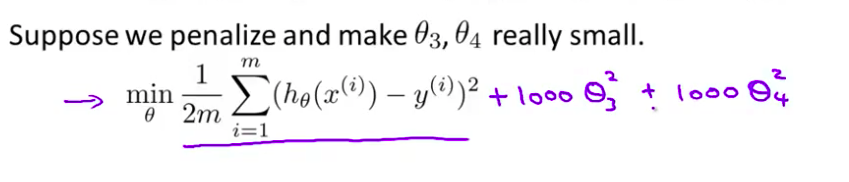• 现在 如果我们要 最小化这个函数 为了使这个 新的代价函数最小化 我们要让 $\theta_3$ 和 $\theta_4$ 尽可能小 对吧？ 因为 如果你有 1000 乘以 $\theta_3$ 这个 新的代价函数将会是很大的 所以 当我们最小化 这个新的函数时 我们将使 $\theta_3$ 的值 接近于0 $\theta_4$ 的值也接近于0 就像我们忽略了 这两个值一样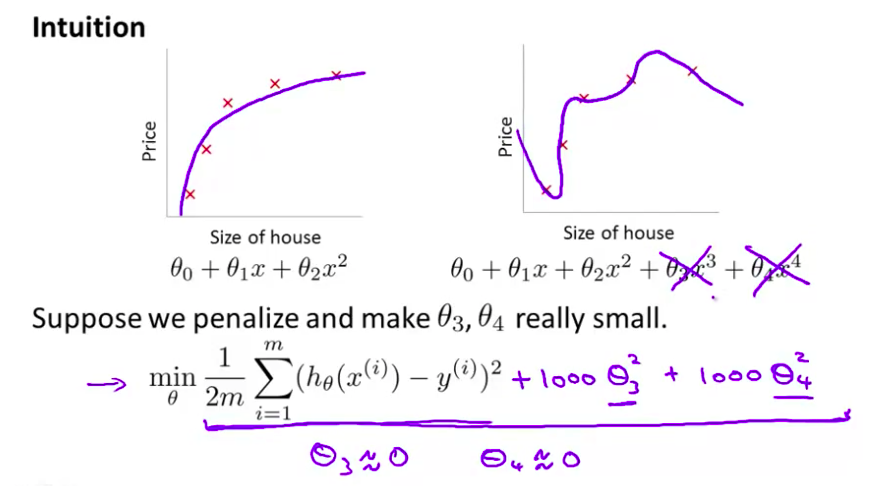• 如果我们做到这一点 如果 $\theta_3$ 和 $\theta_4$ 接近0 那么我们 将得到一个近似的二次函数 所以 我们最终 恰当地拟合了数据 你知道 二次函数加上一些项 这些很小的项 贡献很小 因为 $\theta_3$ $\theta_4$ 它们是非常接近于0的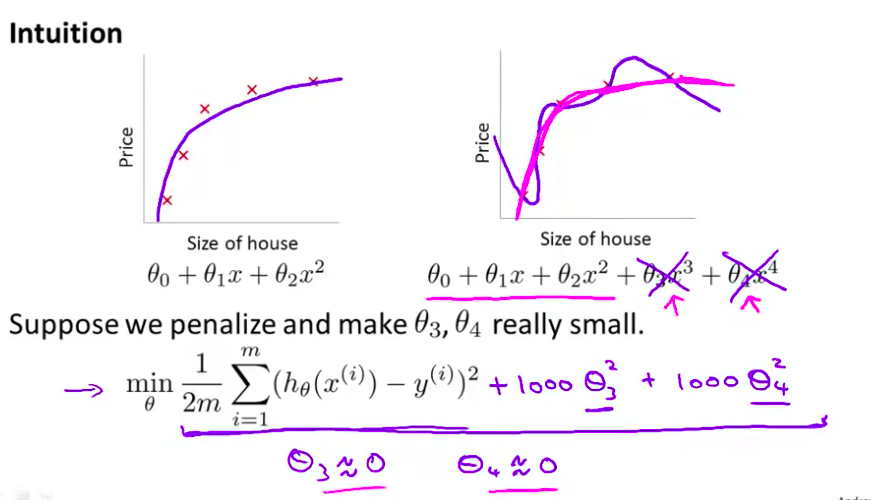• 所以 我们最终得到了 实际上 很好的一个二次函数 因为这是一个 更好的假设 在这个具体的例子中 我们看到了 惩罚这两个 大的参数值的效果 更一般地 这里给出了正规化背后的思路
• 这种思路就是 如果我们 的参数值 对应一个较小值的话 就是说 参数值比较小 那么往往我们会得到一个 形式更简单的假设 所以 我们最后一个例子中 我们惩罚的只是 $\theta_3$ 和 $\theta_4$ 使这两个 值均接近于零 我们得到了一个更简单的假设 也即这个假设大抵上是一个二次函数 但更一般地说 如果我们就像这样 惩罚的其它参数 通常我们 可以把它们都想成是 得到一个更简单的假设 因为你知道 当这些参数越接近这个例子时 假设的结果越接近 一个二次函数 但更一般地 可以表明 这些参数的值越小 通常对应于越光滑的函数 也就是更加简单的函数 因此 就不易发生过拟合的问题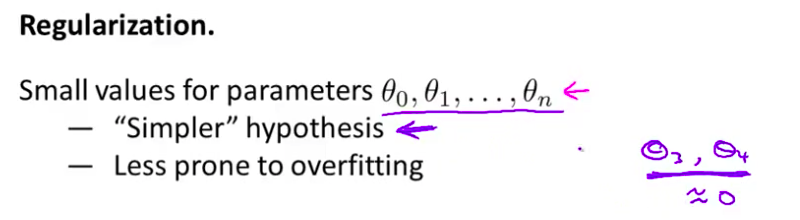• 我知道 为什么要所有的部分参数变小的这些原因 为什么越小的参数对应于一个简单的假设 我知道这些原因 对你来说现在不一定完全理解 但现在解释起来确实比较困难 除非你自己实现一下 自己亲自运行了这部分 但是我希望 这个例子中 使 $\theta_3$ 和 $\theta_4$ 很小 并且这样做 能给我们一个更加简单的 假设 我希望这个例子 有助于解释原因 至少给了 我们一些直观感受 为什么这应该是这样的
• 来让我们看看具体的例子 对于房屋价格预测我们 可能有上百种特征 我们谈到了一些可能的特征 比如说 x1 是房屋的尺寸 x2 是卧室的数目 x3 是房屋的层数等等 那么我们可能就有一百个特征 跟前面的多项式例子不同 我们是不知道的 对吧 我们不知道 θ3 θ4 是高阶多项式的项 所以 如果我们有一个袋子 如果我们有一百个特征 在这个袋子里 我们是很难 提前选出那些 关联度更小的特征的 也就是说如果我们有一百或一百零一个参数 我们不知道 挑选哪一个 我们并不知道 如何选择参数 如何缩小参数的数目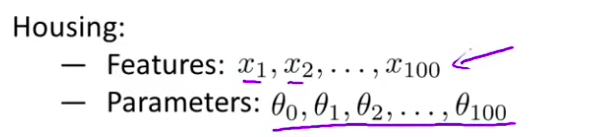• 因此在正规化里 我们要做的事情 就是把我们的 代价函数 这里就是线性回归的代价函数 接下来我来修改这个代价函数 从而 缩小我所有的参数值 因为你知道 我不知道是哪个 哪一个或两个要去缩小 所以我就修改我的 代价函数 在这后面添加一项 就像我们在方括号里的这项 当我添加一个额外的 正则化项的时候 我们收缩了每个 参数 并且因此 我们会使 我们所有的参数 θ1 θ2 θ3 直到 θ100 的值变小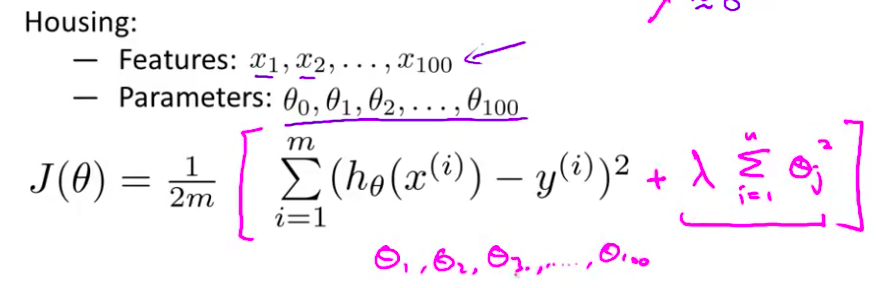• 顺便说一下 按照惯例来讲 我们从第一个这里开始 所以我实际上没有去惩罚 $\theta_0$ 因此 $\theta_0$ 的值是大的 这就是一个约定 从1到 n 的求和 而不是从0到 n 的求和 但其实在实践中 这只会有非常小的差异 无论你是否包括这项 就是 $\theta_0$这项 实际上 结果只有非常小的差异 但是按照惯例 通常情况下我们还是只 从 $\theta_1$ 到 $\theta_{100}$ 进行正规化
• 这里我们写下来 我们的正规化优化目标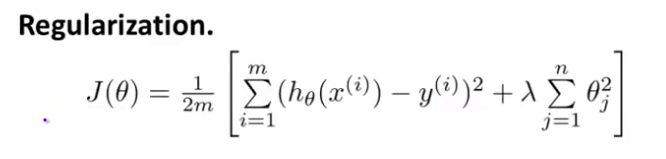• 我们的正规化后的代价函数 就是这样的 $J(\theta)$ 这个项 右边的这项就是一个正则化项 并且 $\lambda$ 在这里我们称做正规化参数 $\lambda$ 要做的就是控制 在两个不同的目标中 的一个平衡关系 第一个目标 第一个需要抓住的目标 就是我们想要训练 使假设更好地拟合训练数据 我们希望假设能够很好的适应训练集 而第二个目标是 我们想要保持参数值较小 这就是第二项的目标 通过正则化目标函数 这就是 $\lambda$ 这个正则化 参数需要控制的 它会这两者之间的平衡 目标就是平衡拟合训练的目的 和 保持参数值较小的目的 从而来保持假设的形式相对简单 来避免过度的拟合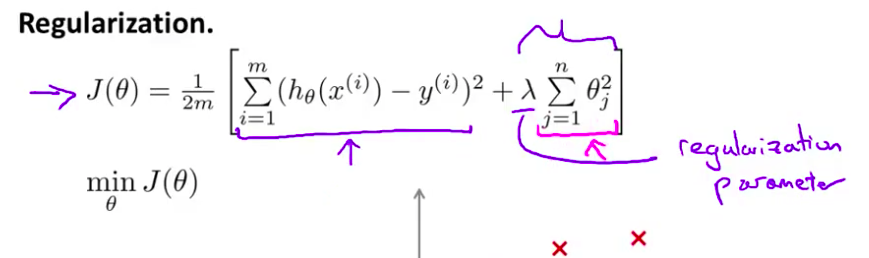• 对于我们的房屋价格预测来说 这个例子 尽管我们之前有 我们已经用非常高的 高阶多项式来拟合 我们将会 得到一个 非常弯曲和复杂的曲线函数 就像这个 如果你还是用高阶多项式拟合 就是用这里所有的多项式特征来拟合的话 但现在我们不这样了 你只需要确保使用了 正规化目标的方法 那么你就可以得到 实际上是一个曲线 但这个曲线不是 一个真正的二次函数 而是更加的流畅和简单 也许就像这条紫红色的曲线一样 那么 你知道的 这样就得到了对于这个数据更好的假设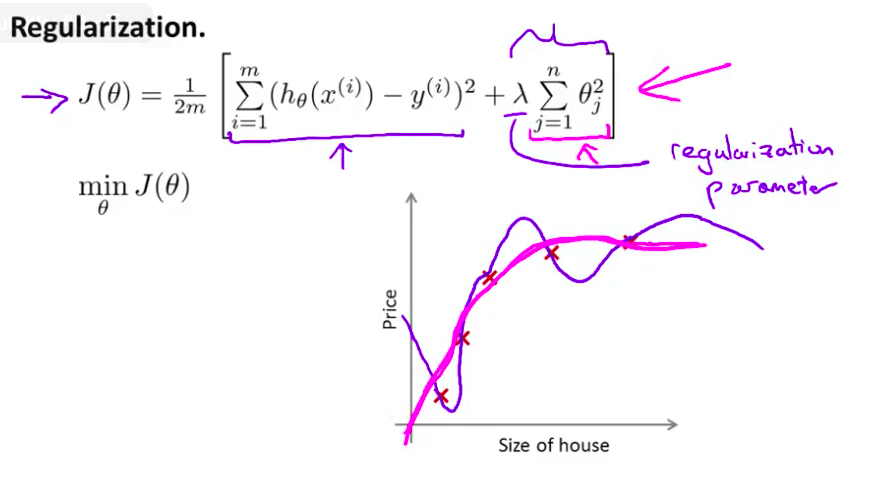• 再一次说明下 我了解这部分有点难以明白 为什么加上 参数的影响可以具有 这种效果 但如果你 亲自实现了正规化 你将能够看到 这种影响的最直观的感受
• 在正规化线性回归中 如果 正规化参数值 被设定为非常大 那么将会发生什么呢？ 我们将会非常大地惩罚 参数$\theta_1$ $\theta_2$ $\theta_3$ $\theta_4$ 也就是说 如果我们的假设是底下的这个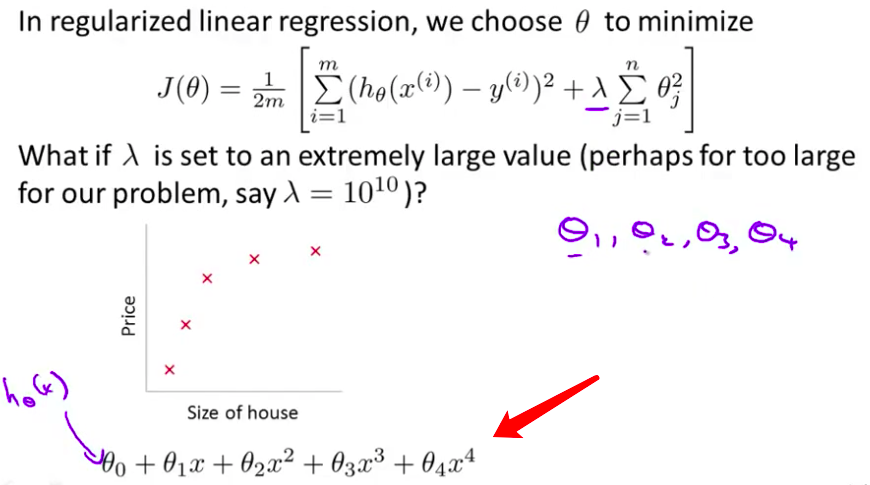• 如果我们最终惩罚 $\theta_1$ $\theta_2$ $\theta_3$ $\theta_4$ 在一个非常大的程度 那么我们 会使所有这些参数接近于零的 对不对？ $\theta_1$ 将接近零 $\theta_2$ 将接近零 $\theta_3$ 和$\theta_4$ 最终也会接近于零 如果我们这么做 那么就是 我们的假设中 相当于去掉了这些项 并且使 我们只是留下了一个简单的假设 这个假设只能表明 那就是 房屋价格 就等于 $\theta_0$ 的值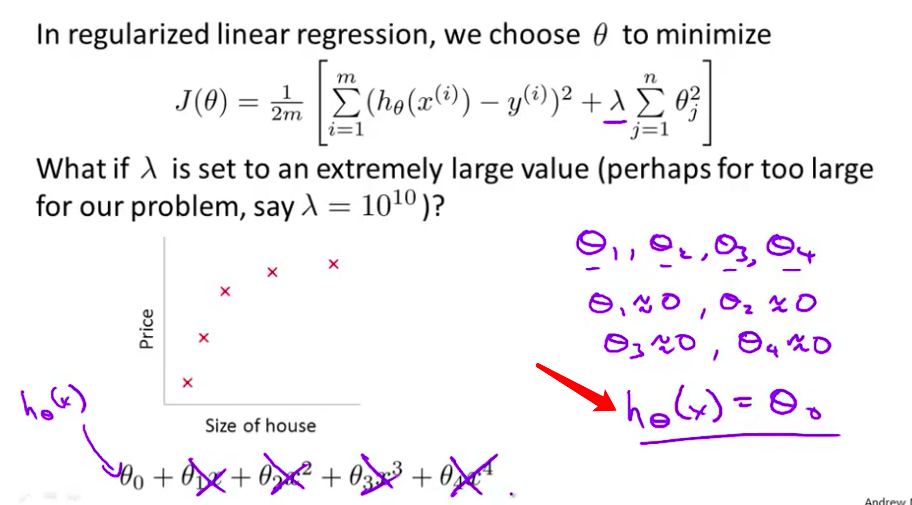• 那就是类似于拟合了 一条水平直线 对于数据来说 这就是一个 欠拟合 (underfitting) 这种情况下这一假设 它是条失败的直线 对于训练集来说 这只是一条平滑直线 它没有任何趋势 它不会去趋向大部分训练样本的任何值 这句话的另​​一种方式来表达就是 这种假设有 过于强烈的”偏见” 或者 过高的偏差 (bais) 认为预测的价格只是 等于 $\theta_0$ 并且 尽管我们的数据集 选择去拟合一条 扁平的直线 仅仅是一条 扁平的水平线 我画得不好 对于数据来说 这只是一条水平线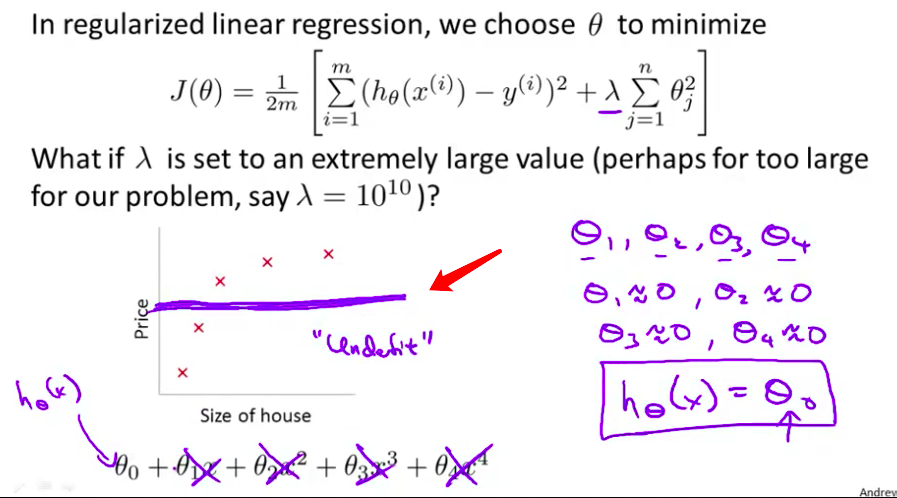• 因此 为了使正则化运作良好 我们应当注意一些方面 应该去选择一个不错的 正则化参数 $\lambda$ 并且当我们以后讲到多重选择时 在后面的课程中 我们将讨论 一种方法 一系列的方法来自动选择 正则化参数 $\lambda$ 所以 这就是高度正则化的思路 回顾一下代价函数 为了使用正则化 在接下来的两段视频中 让我们 把这些概念 应用到 到线性回归和 逻辑回归中去 那么我们就可以让他们 避免过度拟合了

### 小小的总结–代价函数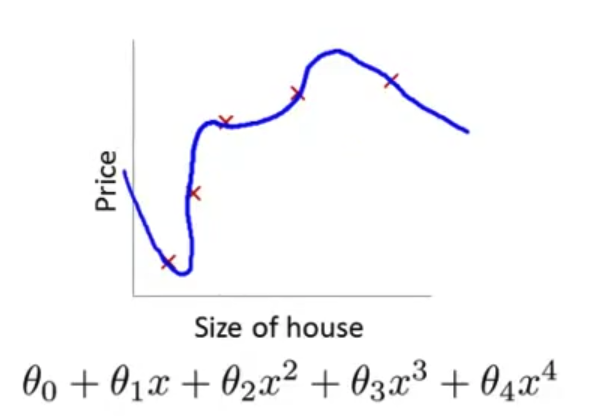${h_\theta}\left( x \right)={\theta_{0}}+{\theta_{1}}{x_{1}}+{\theta_{2}}{x_{2}}+{\theta_{3}}{x_{3}}+{\theta_{4}}{x_{4}}$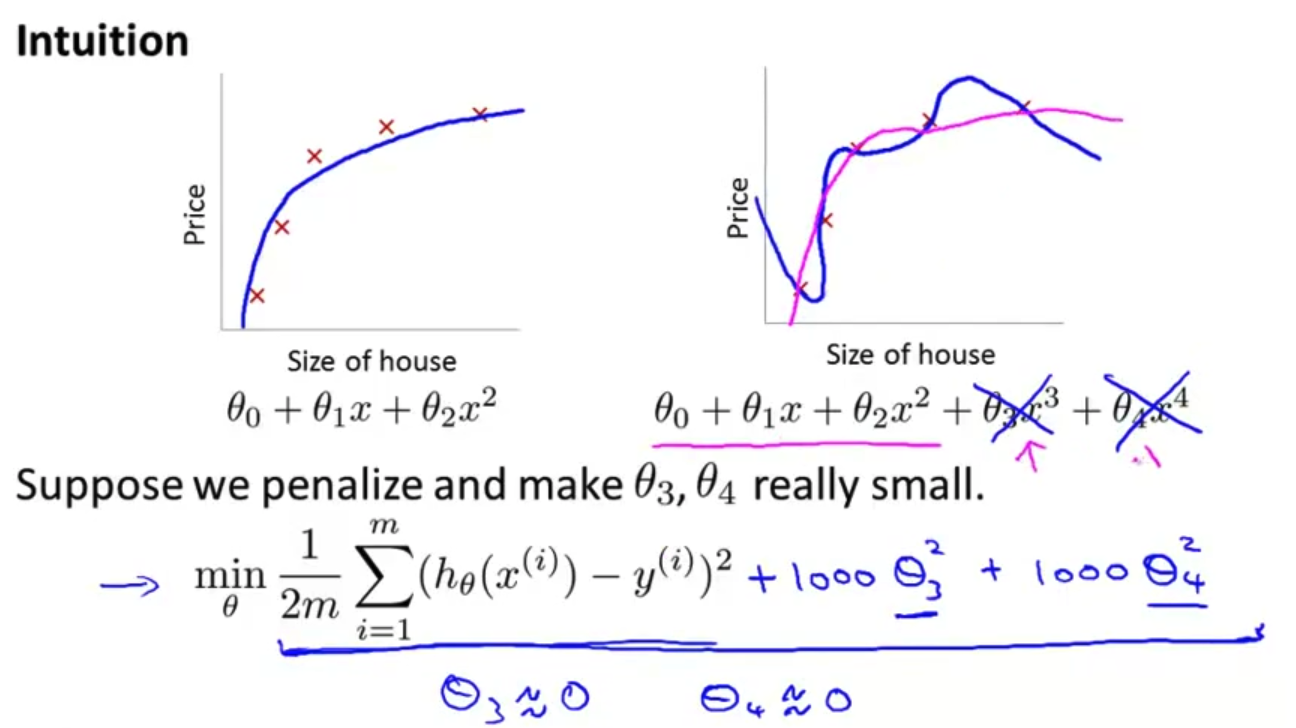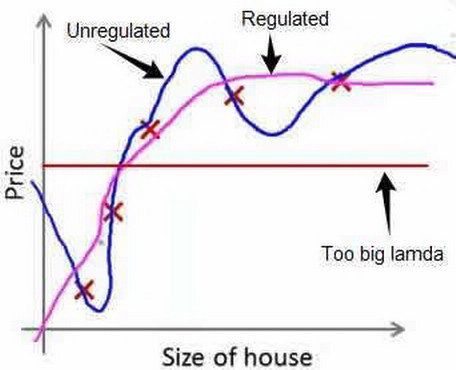## 7.3 正则化线性回归

• 对于线性回归的求解 我们之前 推导了两种学习算法 一种基于梯度下降 一种基于正规方程 在这段视频中 我们将继续学习 这两个算法 并把它们推广 到正则化线性回归中去
• 这是我们上节课推导出的 正则化线性回归的 优化目标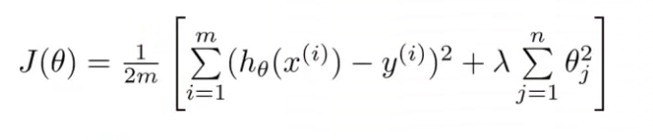• 前面的第一部分是 一般线性回归的目标函数 而现在我们有这个额外的 正则化项 其中 $\lambda$ 是正则化参数 我们想找到参数 $\theta$ 能最小化代价函数 即这个正则化代价函​​数 $J(\theta)$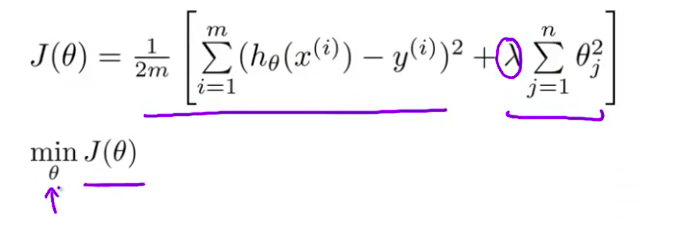• 之前 我们使用 梯度下降求解原来 没有正则项的代价函数 我们用 下面的算法求解常规的 没有正则项的线性回归 我们会如此反复更新 参数 $\theta_j$ 其中 $j=0, 1, 2...n$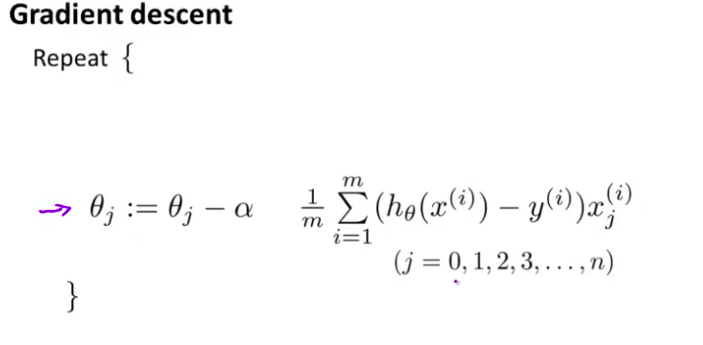• 让我 照这个把 $j=0$ 即 $\theta_0$ 的情况单独写出来 我只是把 $\theta_0$ 的更新 分离出来 剩下的这些参数$\theta_1$, $\theta_2$ 到$\theta_n$的更新 作为另一部分 所以 这样做其实没有什么变化 对吧？ 这只是把 $\theta_0$的更新 和 $\theta_1$ $\theta_2$ 到 $\theta_n$ 的更新分离开来 我这样做的原因是 你可能还记得 对于正则化的线性回归
1:32
我们惩罚参数$\theta_1$ $\theta_2$…一直到 $\theta_n$ 但是我们不惩罚$\theta_0$ 所以 当我们修改这个 正则化线性回归的算法时 我们将对 $\theta_0$ 的方式将有所不同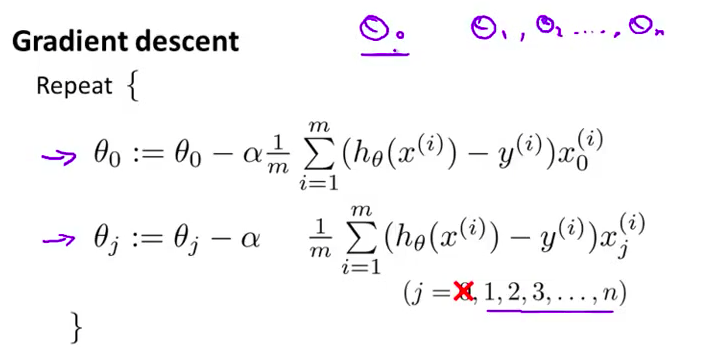• 具体地说 如果我们 要对这个算法进行 修改 并用它 求解正则化的目标函数 我们 需要做的是 把下边的这一项做如下的修改 我们要在这一项上添加一项: $\lambda$ 除以 $m$ 再乘以 $\theta_j$ 如果这样做的话 那么你就有了 用于最小化 正则化代价函数 $J(\theta)$ 的梯度下降算法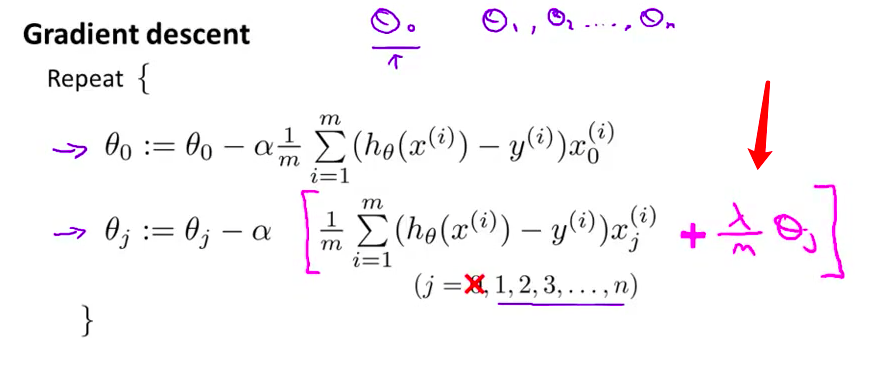• 我不打算用 微积分来证明这一点 但如果你看这一项 方括号里的这一项 如果你知道微积分 应该不难证明它是 $J(\theta)$ 对 $\theta_j$ 的偏导数 这里的 $J(\theta)$ 是用的新定义的形式 它的定义中 包含正则化项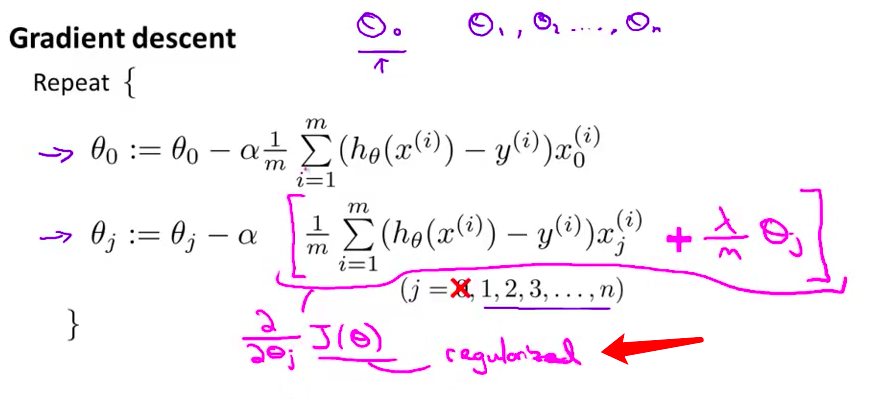• 而另一项 上面的这一项 我用青色的方框 圈出来的这一项 这也一个是偏导数 是 $J(\theta)$对 $\theta_0$ 的偏导数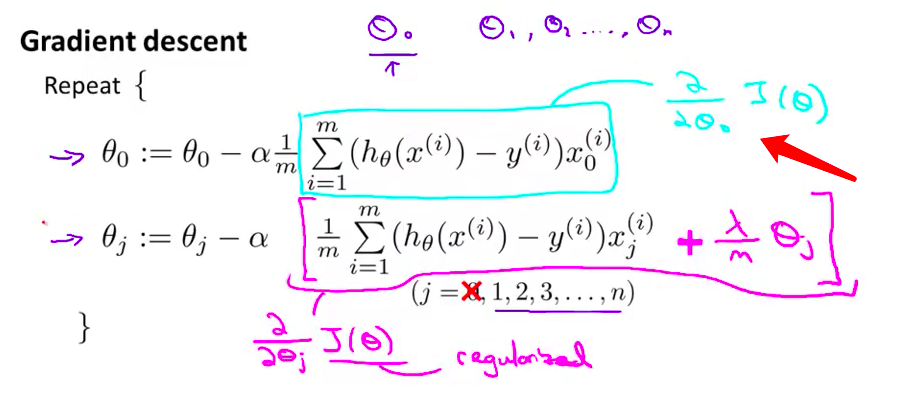• 如果你仔细看 $\theta_j$ 的更新 你会发现一些 有趣的东西 具体来说$\theta_j$的每次更新 都是 $\theta_j$ 自己减去 $\alpha$ 乘以原来的无正则项 然后还有这另外的一项 这一项的大小也取决于 $\theta_j$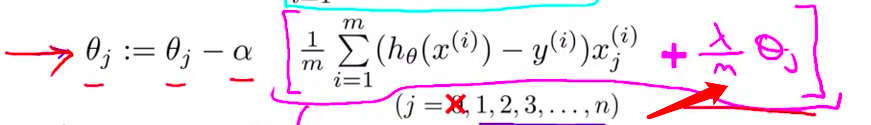• 所以 如果你 把所有这些 取决于 $\theta_j$ 的合在一起的话 可以证明 这个更新 可以等价地写为 如下的形式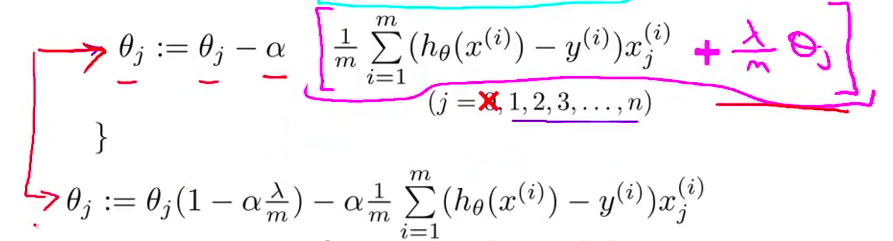• 具体来讲 上面的 $\theta_j$ 对应下面的 $\theta_j$ 乘以括号里的1 而这一项是 $\frac{\lambda}{m}$ 还有一个$\alpha$ 把它们合在一起 所以你最终得到 $\alpha\frac{\lambda}{m}$ 然后合在一起 乘以 $\theta_j$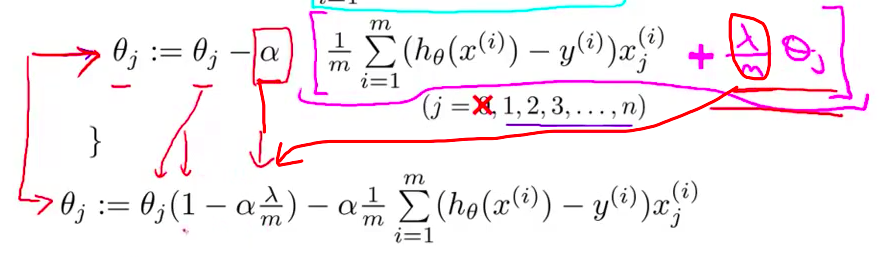• 而这一项 $1-\alpha\frac{\lambda}{m}$ 很有意思 具体来说 这一项 $1-\alpha\frac{\lambda}{m}$ 这一项的值 通常 是一个具体的实数 而且小于1 对吧？由于 $\alpha\frac{\lambda}{m}$ 通常情况下是正的 如果你的学习速率小 而 $m$ 很大的话 $1-\alpha\frac{\lambda}{m}$ 这一项通常是很小的 所以这里的一项 一般来说将是一个比1小一点点的值 所以我们可以把它想成 一个像0.99一样的数字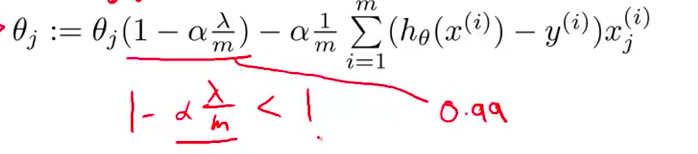• 所以 对 $\theta_j$ 更新的结果 我们可以看作是 被替换为 $\theta_j$ 的0.99倍 也就是 $\theta_j$ 乘以0.99 把 $\theta_j$ 向 0 压缩了一点点 所以这使得 $\theta_j$小了一点 更正式地说 $\theta_j$ 的平方 更小了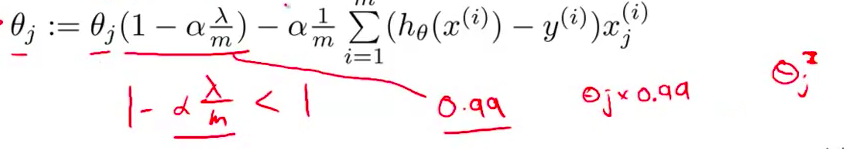• 另外 这一项后边的第二项 这实际上 与我们原来的 梯度下降更新完全一样 跟我们加入了正则项之前一样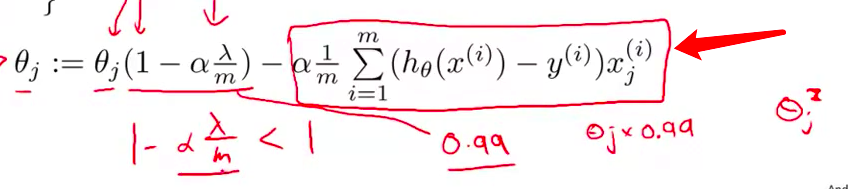• 好的 现在你应该对这个 梯度下降的更新没有疑问了 当我们使用正则化线性回归时 我们需要做的就是 在每一个被正规化的参数 $\theta_j$ 上 乘以了一个 比1小一点点的数字 也就是把参数压缩了一点 然后 我们执行跟以前一样的更新

• 梯度下降只是 我们拟合线性回归模型的两种算法 的其中一个 第二种算法是 使用正规方程 我们的做法 是建立这个 设计矩阵 X 其中每一行 对应于一个单独的训练样本 然后创建了一个向量 y 是一个 m 维的向量 包含了所有训练集里的标签 所以 X 是一个 m × (n+1) 维矩阵 y 是一个 m 维向量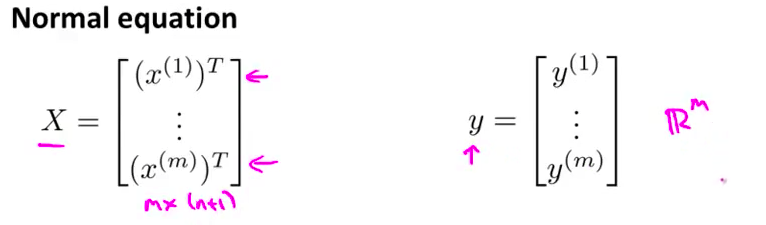• 为了最小化代价函数 $J$ 我们发现 一个办法就是 一个办法就是 让 $\theta$ 等于这个式子 即 X 的转置乘以 X 再对结果取逆 再乘以 X 的转置乘以Y 我在这里留点空间 等下再填满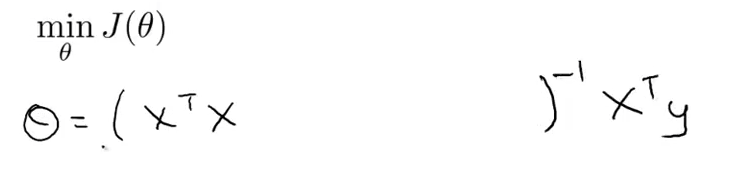• 这个 $\theta$ 的值 其实就是最小化 代价函数 $J(\theta)$ 的$\theta$值 这时的代价函数J(θ)没有正则项 现在如果我们用了是正则化 我们想要得到最小值 我们来看看应该怎么得到
• 推导的方法是 取 $J$ 关于各个参数的偏导数 并令它们 等于0 然后做些 数学推导 你可以 得到这样的一个式子 它使得代价函数最小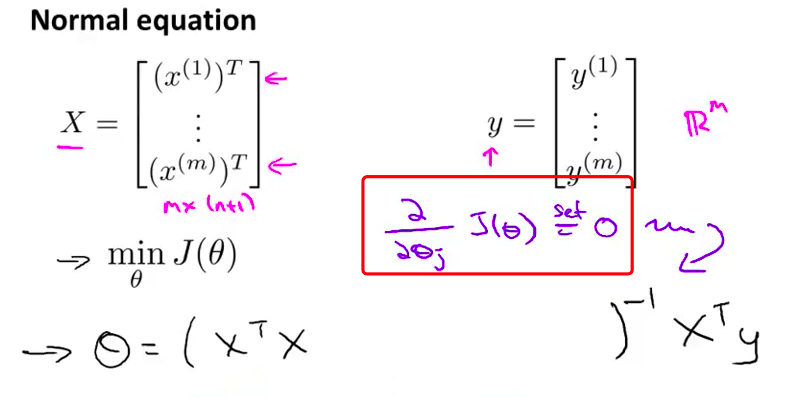• 具体的说 如果你 使用正则化 那么公式要做如下改变 括号里结尾添这样一个矩阵 0 1 1 1 等等 直到最后一行 所以这个东西在这里是 一个矩阵 它的左上角的元素是0 其余对角线元素都是1 剩下的元素也都是 0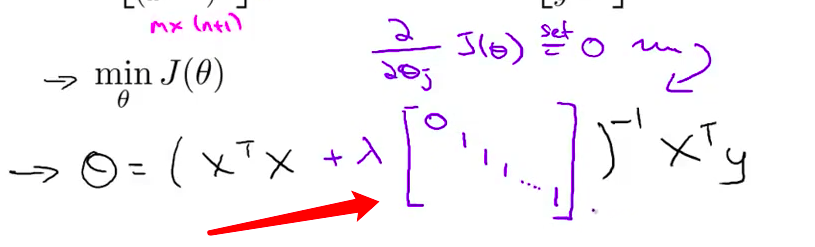• 可以举一个例子 如果 n 等于2 那么这个矩阵 将是一个3 × 3 矩阵 更一般地情况 该矩阵是 一个 (n+1) × (n+1) 维的矩阵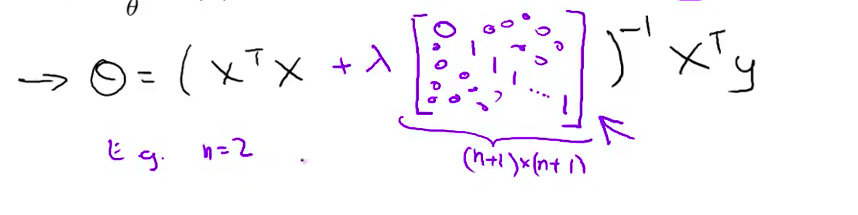• 因此 n 等于2时 矩阵看起来会像这样 左上角是0 然后其他对角线上是1 其余部分都是0 同样地 我不打算对这些作数学推导 坦白说这有点费时耗力 但可以证明 如果你采用新定义的 $J(\theta)$ 包含正则项的目标函数 那么这个计算 $\theta$ 的式子 能使你的 $J(\theta)$ 达到全局最小值

• 所以最后 我想快速地谈一下不可逆性的问题 这部分是比较高阶的内容 所以这一部分还是作为选学 你可以跳过去 或者你也可以听听 如果听不懂的话 也没有关系 之前当我讲正规方程的时候 我们也有一段选学视频 讲不可逆的问题 所以这是另一个选学内容 可以作为上次视频的补充 可以作为上次视频的补充

• 现在考虑 m 即样本总数 小与或等于特征数量 n 如果你的样本数量 比特征数量小的话 那么这个矩阵 X 转置乘以 X 将是 不可逆或奇异的(singluar) 或者用另一种说法是 这个矩阵是退化(degenerate)的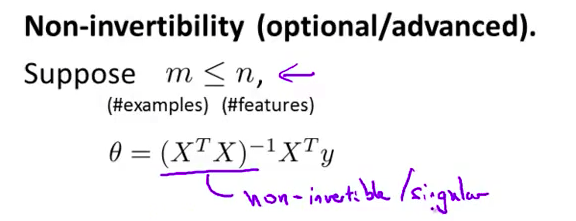• 如果你在 Octave 里运行它 无论如何 你用函数 pinv 取伪逆矩阵 这样计算 理论上方法是正确的 但实际上 你不会得到一个很好的假设 尽管 Ocatve 会 用 pinv 函数 给你一个数值解 看起来还不错 但是 如果你是在一个不同的编程语言中 如果在 Octave 中 你用 inv 来取常规逆 也就是我们要对 X 转置乘以 X 取常规逆 然后在这样的情况下 你会发现 X 转置乘以 X 是奇异的 是不可逆的 即使你在不同的 编程语言里计算 并使用一些 线性代数库 试图计算这个矩阵的逆矩阵 都是不可行的 因为这个矩阵是不可逆的或奇异的
• 幸运的是 正规化也 为我们解决了这个问题 具体地说 只要正则参数是严格大于0的 实际上 可以 证明该矩阵 X 转置 乘以 X 加上 λ 乘以 这里这个矩阵 可以证明 这个矩阵将不是奇异的 即该矩阵将是可逆的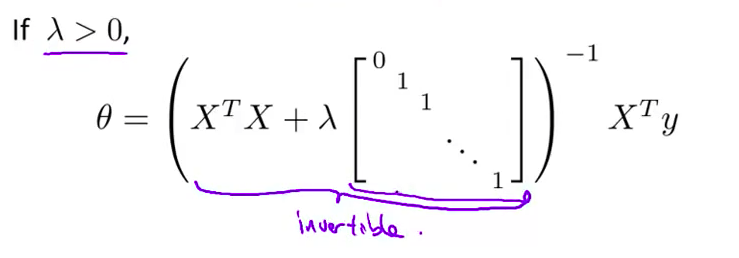• 因此 使用正则化还可以 照顾一些 X 转置乘以 X 不可逆的问题 好的 你现在知道了如何实现正则化线性回归 利用它 你就可以 避免过度拟合 即使你在一个相对较小的训练集里有很多特征 这应该可以让你 在很多问题上更好地运用线性回归 在接下来的视频中 我们将 把这种正则化的想法应用到逻辑回归 这样你就可以 让逻辑回归也避免过度拟合 并让它表现的更好

### 小小的总结–正则化线性回归

$J\left( \theta \right)=\frac{1}{2m}\sum\limits_{i=1}^{m}{[({{({h_\theta}({{x}^{(i)}})-{{y}^{(i)}})}^{2}}+\lambda \sum\limits_{j=1}^{n}{\theta _{j}^{2}})]}$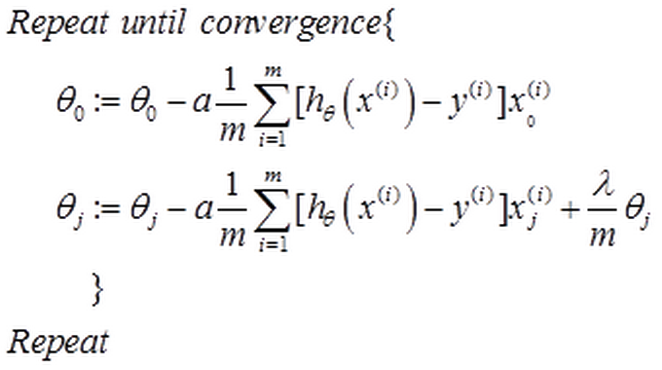${\theta_j}:={\theta_j}(1-a\frac{\lambda }{m})-a\frac{1}{m}\sum\limits_{i=1}^{m}{({h_\theta}({{x}^{(i)}})-{{y}^{(i)}})x_{j}^{\left( i \right)}}$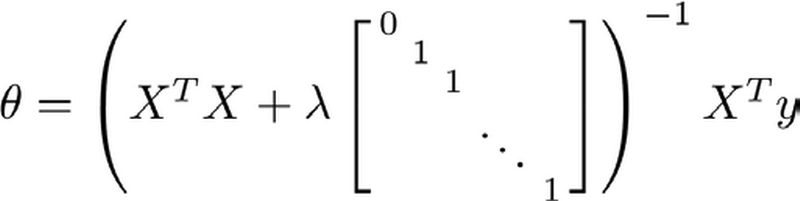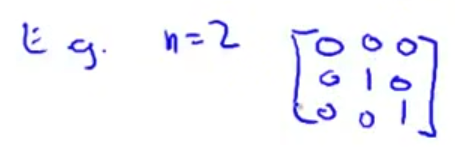## 7.4 正则化的逻辑回归模型

• 针对逻辑回归问题 我们在之前的课程已经学习过两种优化算法 我们首先学习了 使用梯度下降法来优化代价函数 $J(\theta)$ 接下来学习了 更高级的优化算法 这些高级优化算法 需要你自己设计 代价函数 $J(\theta)$ 自己计算导数 在本节课中 我们将展示 如何改进梯度下降法和 高级优化算法 使其能够应用于 正则化的逻辑回归 接下来我们来学习其中的原理
• 在之前的课程中我们注意到 对于逻辑回归问题 有可能会出现过拟合的现象 如果你使用了 类似这样的高阶多项式 g 是 S 型函数 具体来说 最后你会得到这样的结果 分类边界看起来是一个 过于复杂并且 十分扭曲的函数 针对这个训练集 这显然不是一个好的结果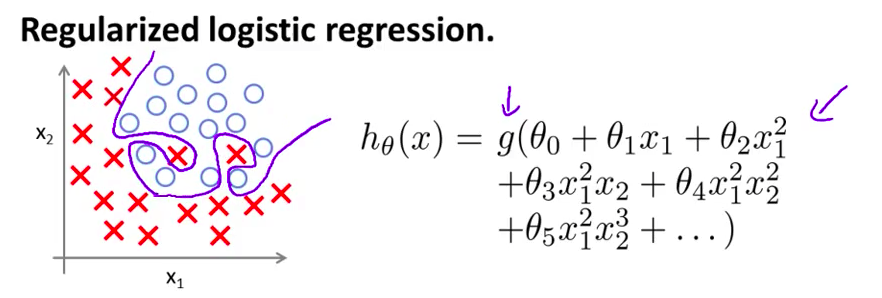• 通常情况下 如果要解决的逻辑回归问题有很多参数 并且又用了过多的多项式项 这些项大部分都是没有必要的 最终都可能出现过拟合的现象
• 这是逻辑回归问题的代价函数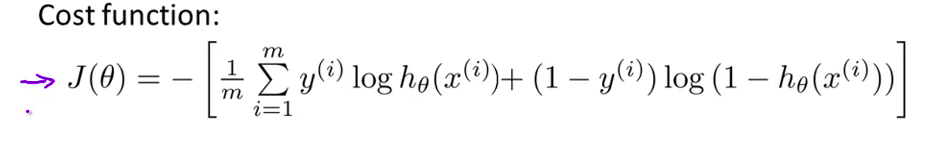• 为了将其修改为正则化形式 我们只需要在后面增加一项 加上 $\frac{\lambda}{2m}$ 再跟过去一样 这个求和将 j 从1开始 而不是从0开始 累积 $\theta_j$的平方 增加的这一项 将惩罚参数 θ1, θ2 等等 一直到 θn 防止这些参数取值过大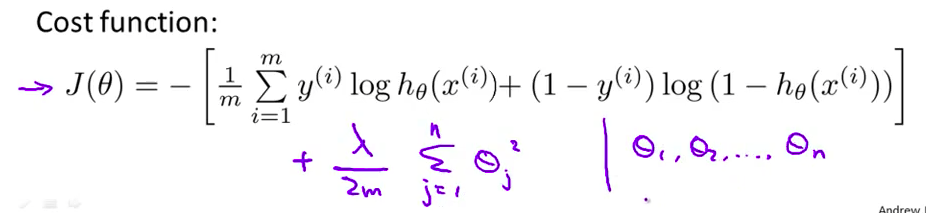• 增加了这一项之后 产生的效果是 即使用有很多参数的 高阶多项式来拟合 只要使用了正则化方法 约束这些参数使其取值很小 你仍有可能得到一条 看起来是这样的分类边界 显然 这条边界更合理地 分开了正样本和负样本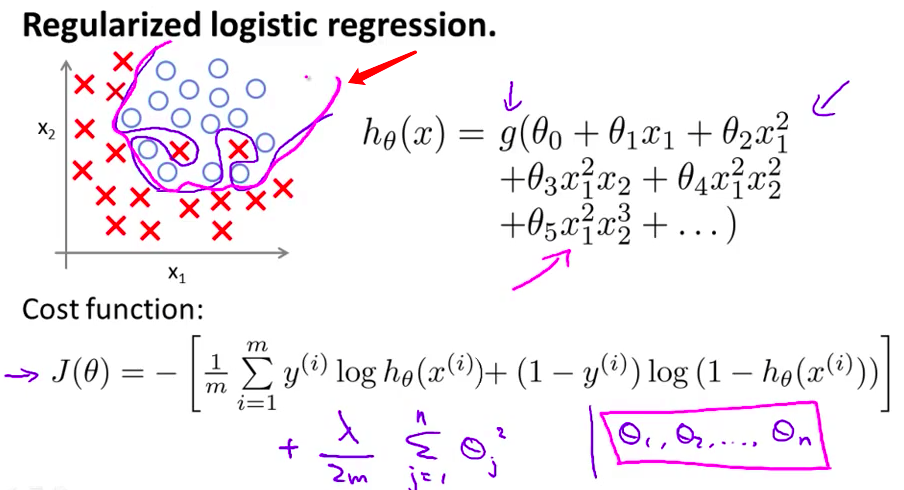• 因此 在使用了正则化方法以后 即使你的问题有很多参数 正则化方法可以帮你 避免过拟合的现象 这到底是怎样实现的呢？
• 首先看看以前学过的梯度下降法 这是我们之前得到的更新式 我们利用这个式子 迭代更新 $\theta_j$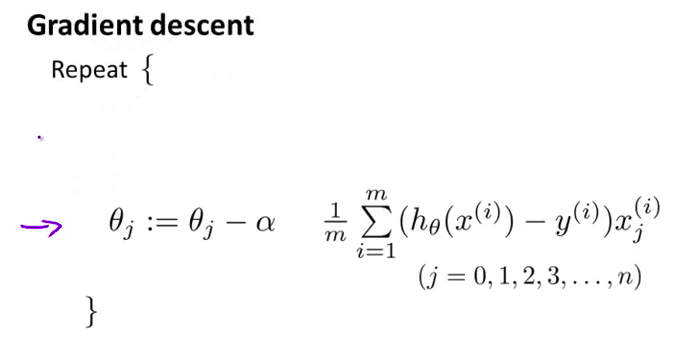• 这一页幻灯片看起来和上一节课的线性回归问题很像 但是这里我将 $\theta_0$ 的更新公式单独写出来 第一行用来更新 $\theta_0$ 第二行用来更新 $\theta_1$ 到 $\theta_n$ ,将 $\theta_0$ 单独处理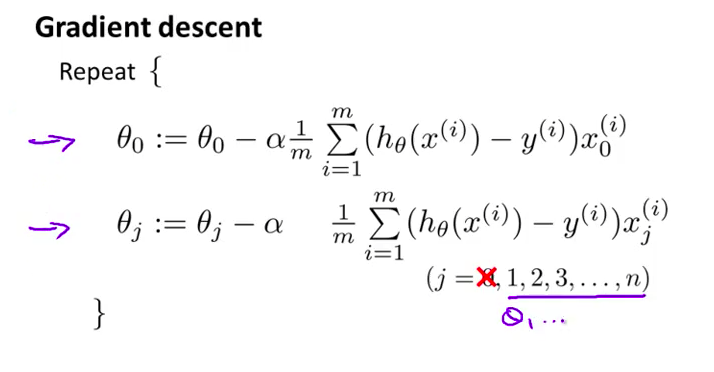• 为了按照 正则化代价函数的形式 来修改算法 接下来的推导 非常类似于 上一节学习过的正则化线性回归 只需要将第二个式子 修改成这样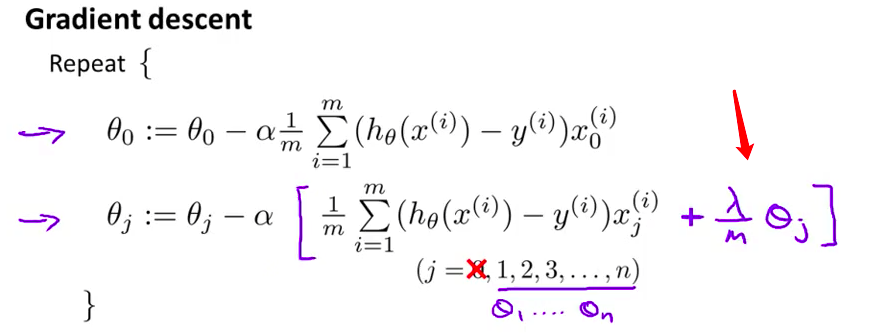• 我们又一次发现 修改后的式子表面上看起来 与上一节的线性回归问题很相似 但是实质上这与 我们上节学过的算法并不一样 因为现在的假设 h(x) 是按照下面式子定义的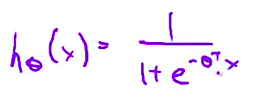• 这与上一节正则化线性回归算法 中的定义并不一样 由于假设的不同 我写下的迭代公式 只是表面上看起来很像 上一节学过的 正则化线性回归问题中的梯度下降算法
• 总结一下 方括号中的这一项是 新的代价函数 $J(\theta)$ 关于 $\theta_j$ 的偏导数 这里的 $J(\theta)$ 是我们在上一页幻灯片中 定义的 使用了正则化的代价函数• 以上就是正则化逻辑回归问题的梯度下降算法 接下来我们讨论 如何在更高级的优化算法中 使用同样的 正则化技术 提醒一下 对于这些高级算法 我们需要自己定义 costFuntion 函数 这个函数有一个输入参数 向量 theta 的内容是这样的 我们的参数索引依然从0开始 即 θ0 到 θn 但是由于 Octave 中 向量索引是从1开始 我们的参数是从 θ0 到 θn 在 Octave 里 是从 theta(1) 开始标号的 而 θ1 将被记为 theta(2) 以此类推 直到 θn 被记为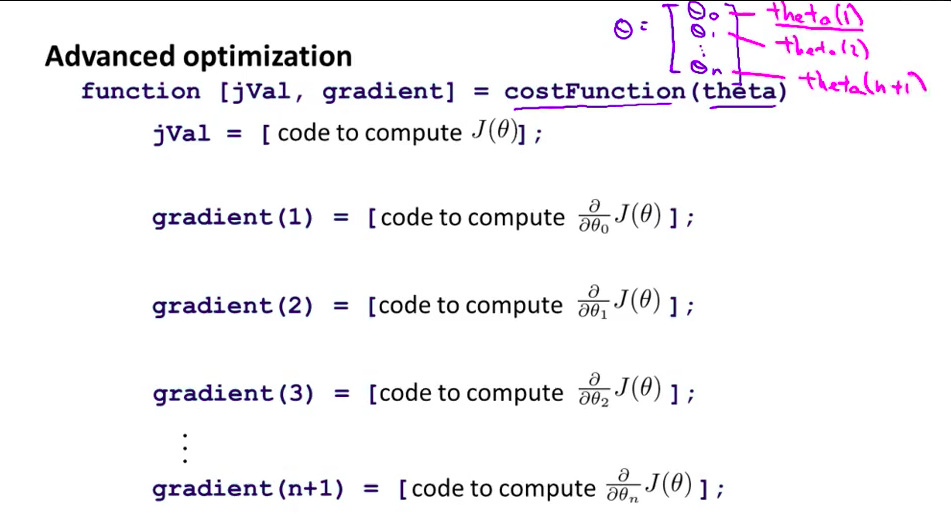• 而我们需要做的 就是将这个自定义代价函数 这个 costFunction 函数 代入到我们之前学过的 fminunc函数中 括号里面是 @costFunction 将 @costFunction 作为参数代进去 等等 fminunc返回的是 函数 costFunction 在无约束条件下的最小值
• 因此 这个式子 将求得代价函数的最小值 因此 costFunction 函数 有两个返回值 第一个是 jVal 为此 我们要在这里 补充代码 来计算代价函数 J(θ)
• 由于我们在这使用的是正则化逻辑回归 因此 代价函数 J(θ) 也相应需要改变 具体来说 代价函数需要 增加这一正则化项 因此 当你在计算 J(θ) 时 需要确保包含了最后这一项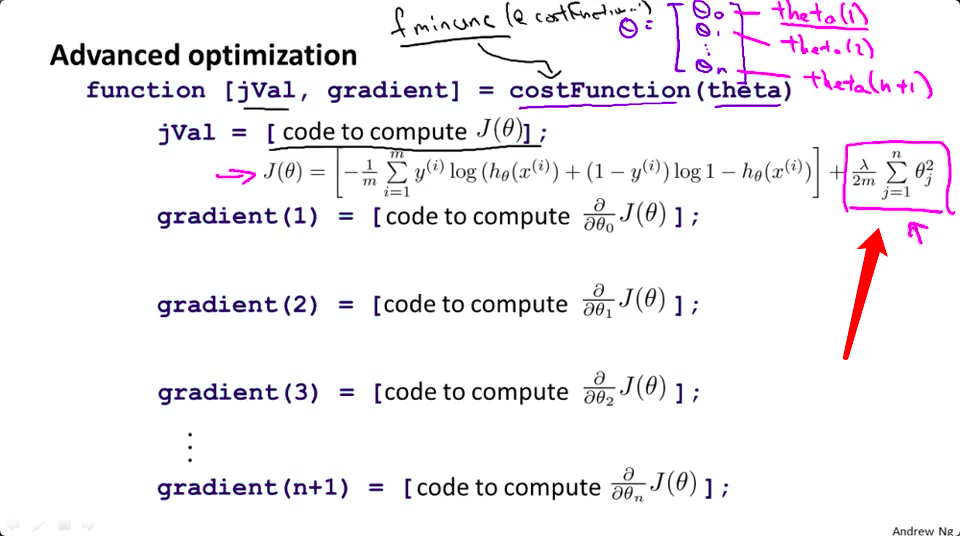• 另外 代价函数的 另一项返回值是 对应的梯度导数 梯度的第一个元素 gradient(1) 就等于 J(θ) 关于 θ0 的偏导数 梯度的第二个元素按照这个式子计算 剩余元素以此类推 再次强调 向量元素索引是从1开始 这是因为 Octave 的向量索引 就是从1开始的
• 再来总结一下 首先看第一个公式 在之前的课程中 我们已经计算过它等于这个式子 这个式子没有变化 因为相比没有正则化的版本 J(θ) 关于 θ0 的偏导数不会改变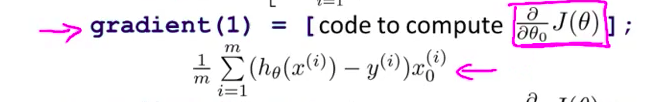• 但是其他的公式确实有变化 以 θ1 的偏导数为例 在之前的课程里我们也计算过这一项 它等于这个式子 加上 λ 除以 m 再乘以 θ1 注意要确保这段代码编写正确 建议在这里添加括号 防止求和符号的作用域扩大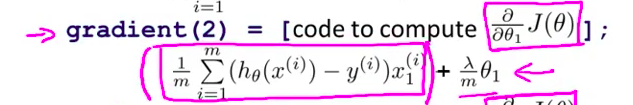• 类似的 再来看这个式子 相比于之前的幻灯片 这里多了额外的一项 这就是正则化后的 梯度计算方法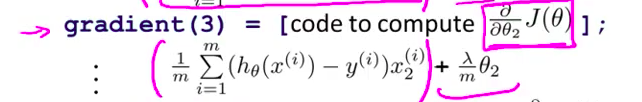• 当你自己定义了 costFunction 函数 并将其传递到 fminuc 或者其他类似的高级优化函数中 就可以求出 这个新的正则化代价函数的极小值 而返回的参数值 即是对应的 逻辑回归问题的正则化解 讲到这里 你应该已经学会了 解决正则化逻辑回归问题的方法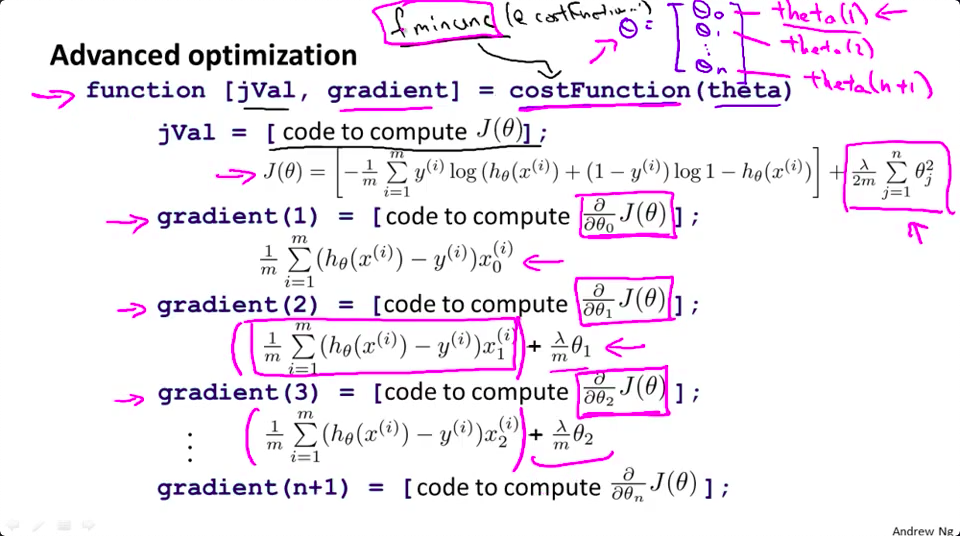• 你知道吗 我住在硅谷 当我在硅谷晃悠时 我看到许多工程师 运用机器学习算法 给他们公司挣来了很多金子 课讲到这里 大家对机器学习算法可能还只是略懂 但是一旦你精通了 线性回归、高级优化算法 和正则化技术 坦率地说 你对机器学习的理解 可能已经比许多工程师深入了 现在 你已经有了 丰富的机器学习知识 目测比那些硅谷工程师还厉害 而那些工程师都混得还不错 给他们公司挣了大钱 你懂的 或者用机器学习算法来做产品 所以 恭喜你 你已经历练得差不多了 已经具备足够的知识 足够将这些算法 用于解决实际问题 所以你可以小小的骄傲一下了 但是 我还是有很多可以教你们的 我还是有很多可以教你们的 接下来的课程中 我们将学习 一个非常强大的非线性分类器 无论是线性回归问题 还是逻辑回归问题 都可以构造多项式来解决 但是 你将逐渐发现还有 更强大的非线性分类器 可以用来解决多项式回归问题 在下一节课 我将向大家介绍它们 你将学会 比你现在解决问题的方法 强大N倍的学习算法

### 小小的总结–正则化的逻辑回归模型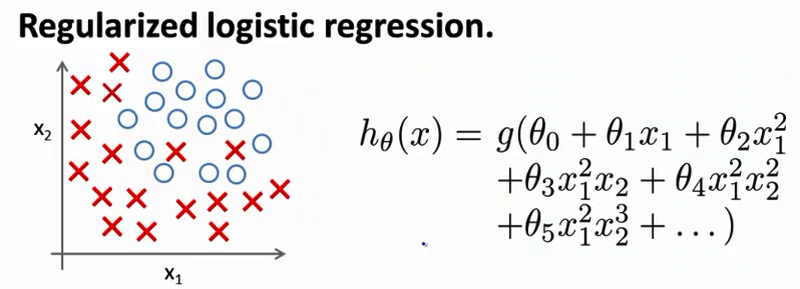$J\left( \theta \right)=\frac{1}{m}\sum\limits_{i=1}^{m}{[-{{y}^{(i)}}\log \left( {h_\theta}\left( {{x}^{(i)}} \right) \right)-\left( 1-{{y}^{(i)}} \right)\log \left( 1-{h_\theta}\left( {{x}^{(i)}} \right) \right)]}+\frac{\lambda }{2m}\sum\limits_{j=1}^{n}{\theta _{j}^{2}}$

Python代码：

import numpy as np

def costReg(theta, X, y, learningRate):
theta = np.matrix(theta)
X = np.matrix(X)
y = np.matrix(y)
first = np.multiply(-y, np.log(sigmoid(X*theta.T)))
second = np.multiply((1 - y), np.log(1 - sigmoid(X*theta.T)))
reg = (learningRate / (2 * len(X))* np.sum(np.power(theta[:,1:theta.shape],2))
return np.sum(first - second) / (len(X)) + reg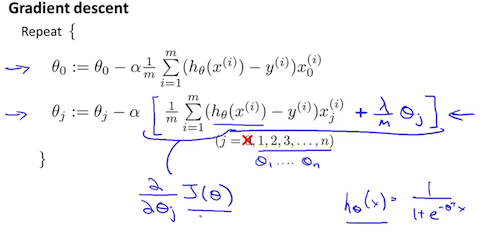Octave 中，我们依旧可以用 fminuc 函数来求解代价函数最小化的参数，值得注意的是参数${\theta_{0}}$的更新规则与其他情况不同。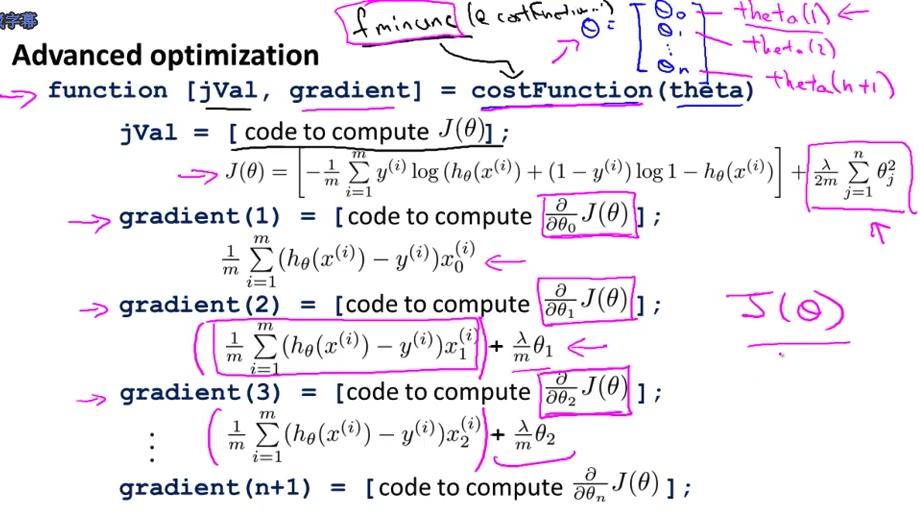1. 虽然正则化的逻辑回归中的梯度下降和正则化的线性回归中的表达式看起来一样，但由于两者的${h_\theta}\left( x \right)$不同所以还是有很大差别。

2. ${\theta_{0}}$不参与其中的任何一个正则化。

转载规则

《第七章-正则化-Regularization》 冯文华 采用 知识共享署名 4.0 国际许可协议 进行许可。
上一篇第八章-神经网络-表述(Neural-Networks-Representation)

2019-02-12第六章-逻辑回归(Logistic-Regression)

2019-02-11
目录